Want to share your content on R-bloggers? click here if you have a blog, or here if you don't.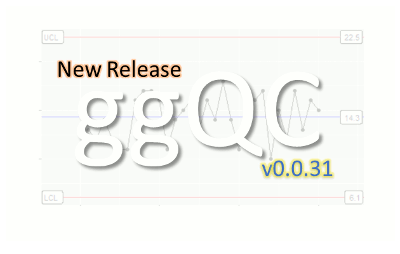The ggQC package is a quality control extension for ggplot. Use it to create XmR, XbarR, C and many other highly customizable Control Charts. Additional statistical process control functions include Shewart violation checks as well as capability analysis. If your process is running smoothly, visualize the potential impacted of your next process improvement with a Pareto chart. To learn more, read on!

To get started with ggQC, install it from CRAN by running the following code:

```install.packages("ggQC")
```

### ggQC Control Charts

Control charts are a great way to monitor process outputs, drive improvement, and evaluate measurement systems. The types of control chart types supported by ggQC include:

The process for building control charts with ggQC is simple. First, load the ggQC and ggplot2 libraries. Next, load your data into R. Your data should be in long-form. The data set below provides an example of long form data if you're not familiar with the term.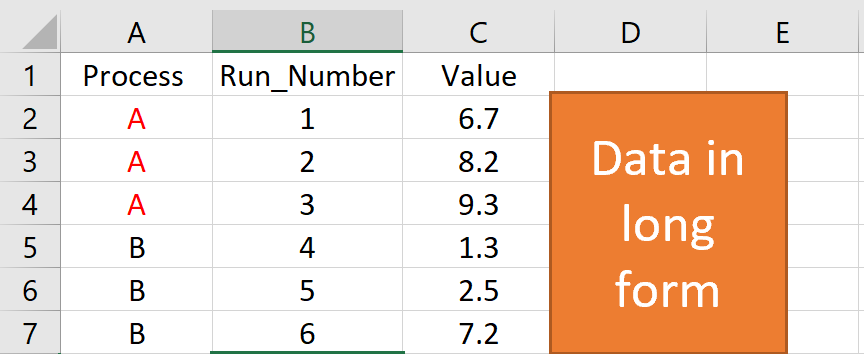Finally, make your control chart using standard ggplot layer-by-layer syntax and the stat_QC() command. The example code below, shows how all these steps come together to make an XmR plot.

```### Load the Needed Libraries
library(ggplot2)
library(ggQC)

set.seed(5555)
Process_Data <-
data.frame(
Process=rep(c("A"), each = 30), #Process A
Run_Number=c(1:30),             #Run Order
Value = c(rnorm(n = 30, mean = 30.5, sd = 1)) #Process A Random Data
)

### Make the plot
XmR_Plot <-
ggplot(Process_Data, aes(x = Run_Number, y = Value)) + #init ggplot
geom_point() + geom_line() + # add the points and lines
stat_QC(method = "XmR",      # specify QC charting method
auto.label = T,      # Use Autolabels
label.digits = 2,    # Use two digit in the label
show.1n2.sigma = T   # Show 1 and two sigma lines
) +
scale_x_continuous(expand =  expand_scale(mult = .15))  # Pad the x-axis

### Draw the plot - Done
XmR_Plot
```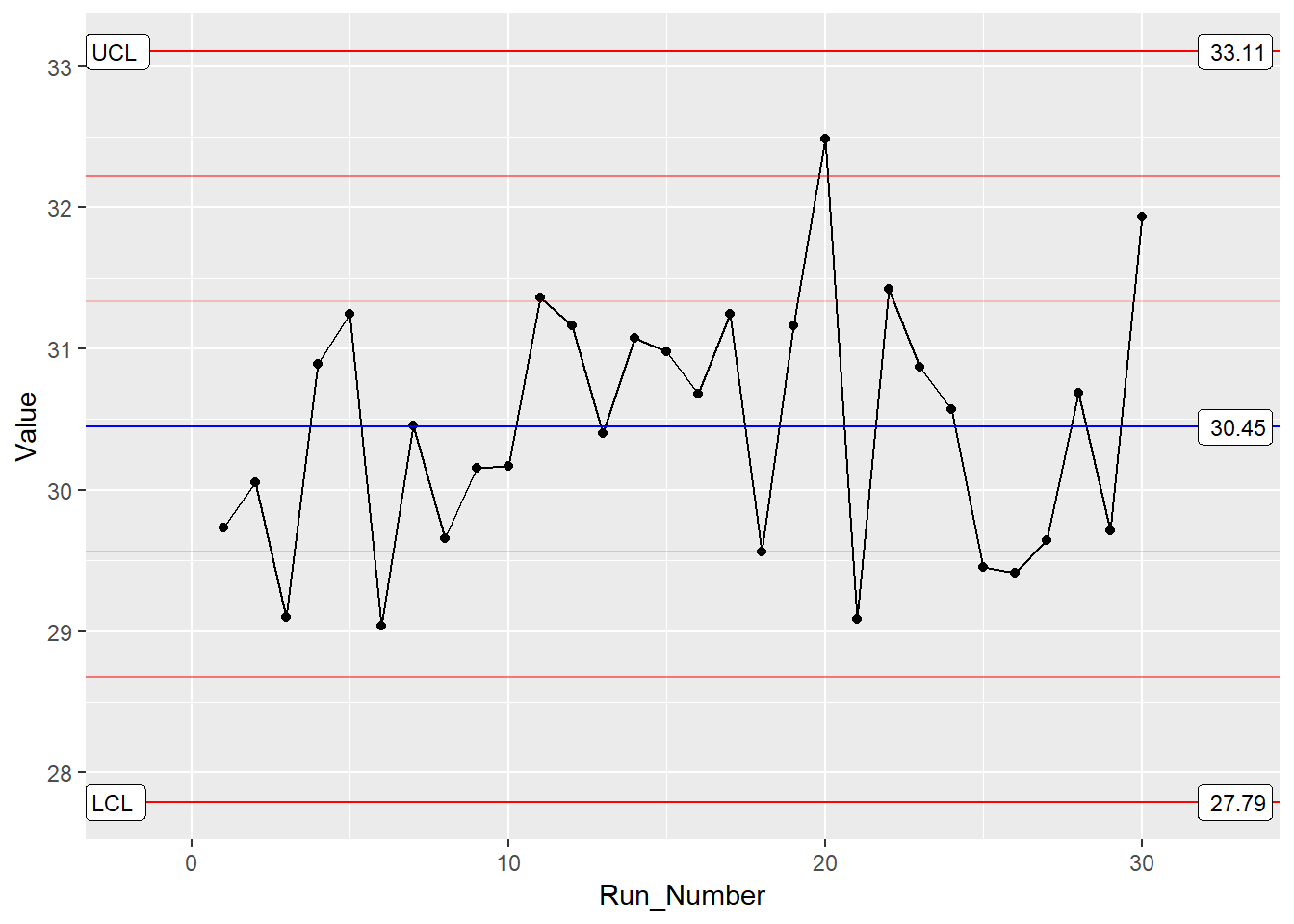By building upon the ggplot framework, you get a high level of control over the plot details such as points and lines etc. In addition, if you want to put XmR and mR data on the same plot, you can. Just make multiple calls to the stat_QC() command, as shown below.

```### Two stat_QC calls
XmR_Plot <-
ggplot(Process_Data, aes(x = Run_Number, y = Value)) + #init ggplot
geom_point() + geom_line() + #add the points and lines
stat_QC(method = "XmR", #specify QC charting method
auto.label = T, # Use Autolabels
label.digits = 2, #Use two digit in the label
show.1n2.sigma = T  #Show 1 and two sigma lines
) +
stat_QC(method="mR") +
scale_x_continuous(expand =  expand_scale(mult = .15))  # Pad the x-axis

### Draw the plot - Done
XmR_Plot
```For more control chart examples, checkout the docs, HOWTOs, and Vignettes at rcontrolcharts.com.

### Violation Analysis

To check for out of control data in your process, use the stat_qc_violations() command. When you run this command, your data is checked against the following 4 Shewart violation rules:

• Same Side: 8 or more consecutive, same-side points
• 1 Sigma: 4 or more consecutive, same-side points exceeding 1 sigma
• 2 Sigma: 2 or more consecutive, same-side points exceeding 2 sigma
• 3 Sigma: any points exceeding 3 sigma

This next bit of code demonstrates a violation analysis with the stat_qc_violation() command using process data from the previous section.

```#Uses the same data as previous example.

QC_Violations <-
ggplot(Process_Data, aes(x = Run_Number, y = Value)) + #init ggplot
stat_qc_violations(method = "XmR"
#show.facets = 4 #if you just want facet 4
)
QC_Violations
```

After executing the code, you should see a plot with 4 facets - one for each Shewart rule. If you only want to see the 4th facet, set show.facets = 4. Other settings such as show.facets = c(2, 4) will show 1 and 3 sigma violations, only.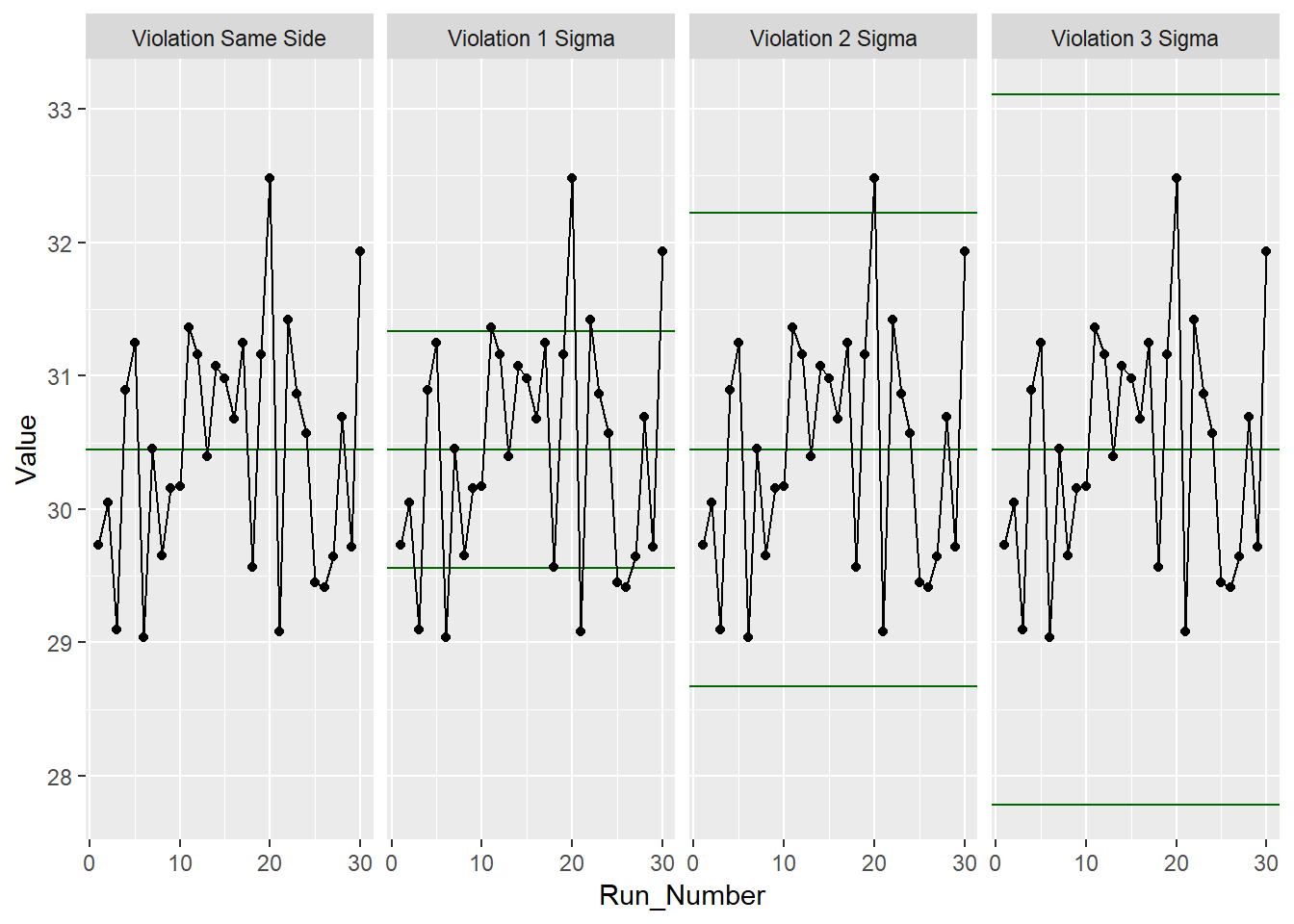For our test data, none of the standard 4 Shewart violations were observed. Awesome! Next, we’ll look at doing a capability analysis with ggQC.

### Capability Analysis

In the previous sections, you learned how to make a control chart with ggQC and check for violations. Here you'll learn how to do a basic capability analysis (Cp, Cpk, Pp, Ppk etc.). For this, we assume the customer has a lower specification limit (LSL) and upper specification limit (USL) of 25 and 37, respectively. With these specifications and the stat_QC_Capability() command, you can do a graphical capability analysis in just a few simple lines of code:

```# Uses the same data as the first example
CapabilityAnaylsis <-
ggplot(Process_Data, aes(x = Value)) + #init ggplot
geom_histogram(binwidth = .75, color="purple") + #make the histogram
stat_QC_Capability(
LSL=25, USL=37,                    #Specify LSL and USL
show.cap.summary = c("Cp", "Cpk"), #selected summary
digits = 2,                        #report two digits
method="XmR") +                    #Use the XmR method
scale_x_continuous(expand =  expand_scale(mult = c(0.15,.65))) #pad the X-axis

#plot the graph
CapabilityAnaylsis
```

To adjust the capability metrics displayed on the plot, provide the show.cap.summary argument with a vector of desired metrics. Metrics available include:

• TOL: Tolerance in Sigma Units (USL-LSL)/sigma
• DNS: Distance to Nearest Specification Limit in Sigma Units
• Cp: Cp (Within sample elbow-room metric)
• Cpk: Cpk (Within sample centering metric)
• Pp: Pp (Between sample elbow-room metric)
• Ppk: Ppk (Between sample centering metric)
• LCL: Lower Control Limit
• X: Process Center
• UCL: Upper Control Limit
• Sig: Sigma from control charts

The order given in the vector is the order displayed on the chart. In this case, only Cp and Cpk were selected, as shown below.Cool! Looks like the process is in good shape. To see more examples of capability analysis, checkout the ggQC documentation and examples on stat_QC_Capability. stat_QC_Capability is also compatible with ggplot faceting. Note that XbarR capability charts are specified slightly different than XmR.

### Pareto Analysis

Alright, so your processes are in control. However, you know your process has bottlenecks. Where should you start? One way to help plan your attack is with a Pareto analysis. Suppose you have the following data showing how long several typical process steps take.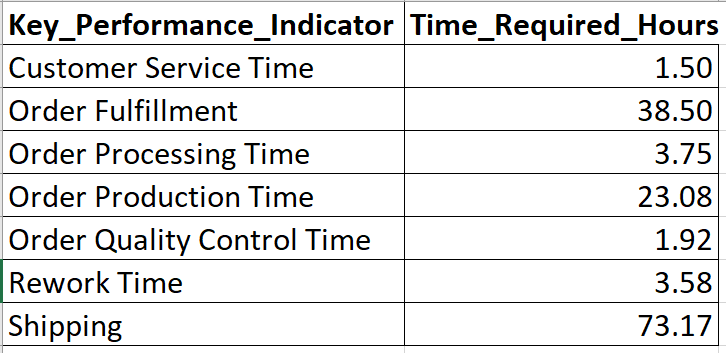To generate a Pareto chart, load the data, initialize ggplot, and let the stat_pareto() command do the rest.

```#load your data
Data4Pareto <- data.frame(
KPI = c("Customer Service Time", "Order Fulfillment", "Order Processing Time",
"Order Production Time", "Order Quality Control Time", "Rework Time",
"Shipping"),
Time = c(1.50, 38.50, 3.75, 23.08, 1.92, 3.58, 73.17)
)

#make the plot
ggplot(Data4Pareto, aes(x=KPI, y=Time)) +
stat_pareto(point.color = "red",
point.size = 3,
line.color = "black",
bars.fill = c("blue", "orange")
) +
theme(axis.text.x = element_text(angle = 90, hjust = 1, vjust=0.5))

#done
```Looks like our next improvement project will focus on either shipping or order fulfillment. Good Luck!

### Summary

Building control charts with ggQC is quick and easy, especially if you're already familiar with ggplot. Like other ggplot graphs, ggQC control charts support faceting and are built up layer-by-layer. If you need to make a complicated chart, go ahead. You can add as many stat_QC calls as you like (see XbarR_Vignette). In addition to control charting, ggQC allows you to run Pareto, capability, and Shewart violation analysis. To learn more, please visit rcontrolcharts.com